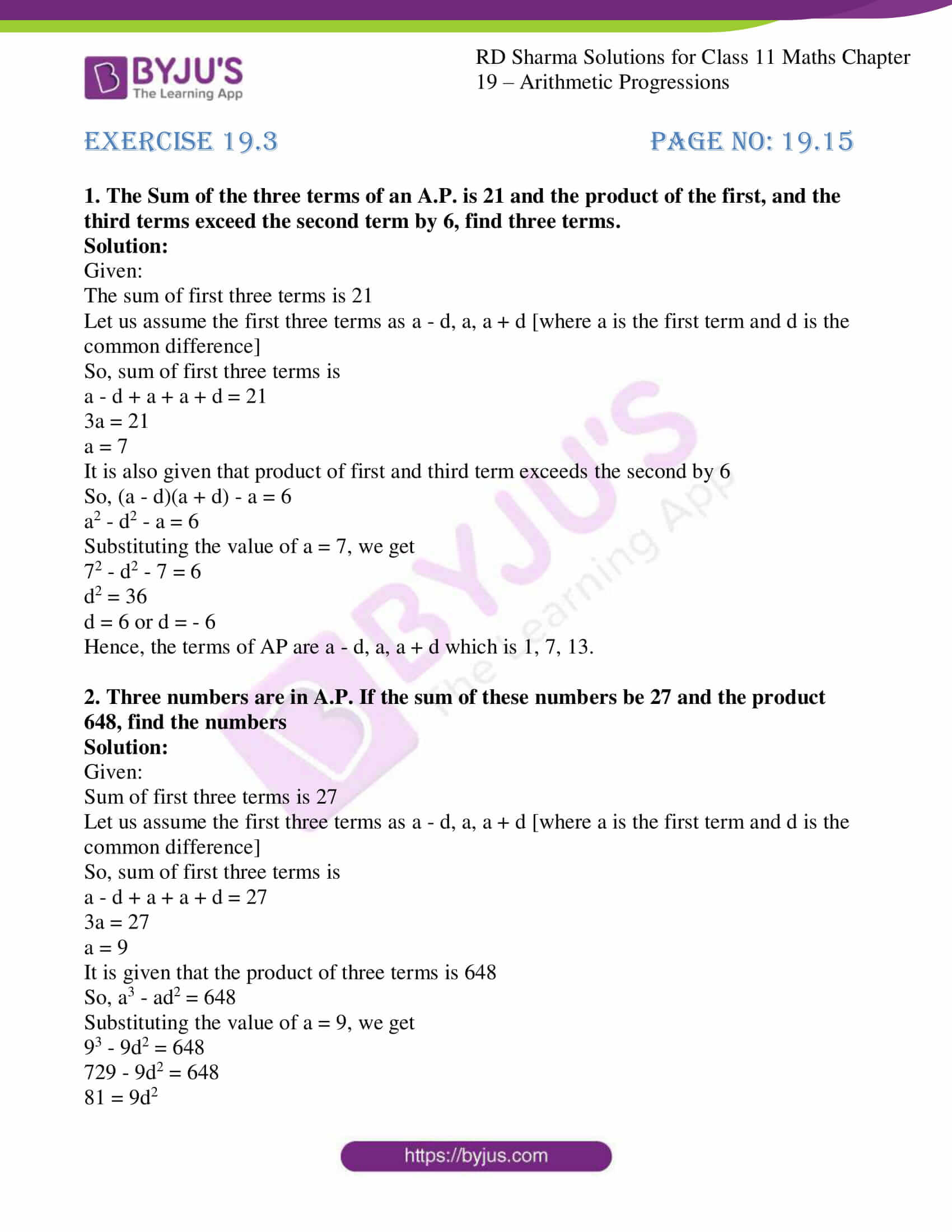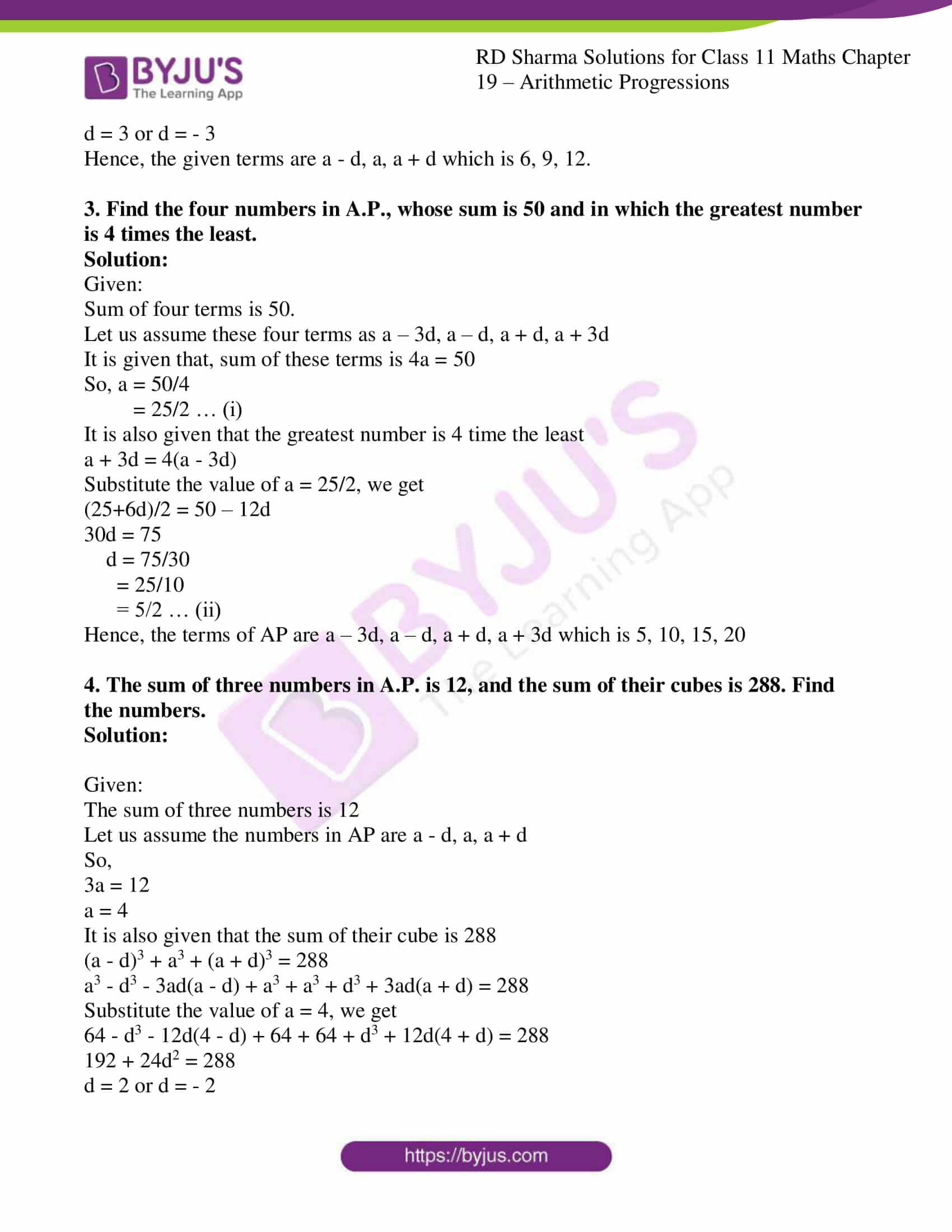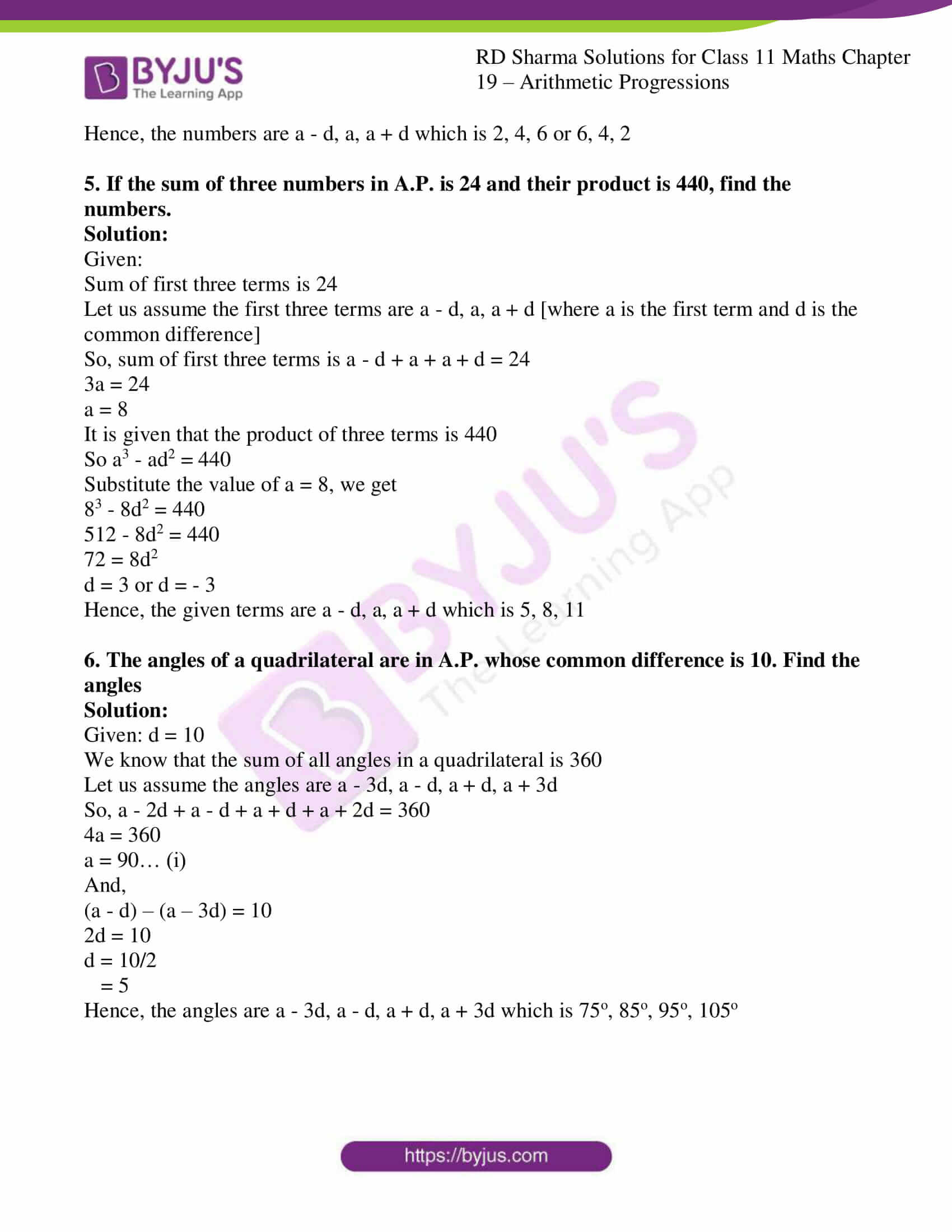# RD Sharma Solutions for Class 11 Chapter 19 - Arithmetic Progressions Exercise 19.3

Sometimes we require a certain number of terms in A.P. there are ways of selecting terms, which are very convenient. Exercise 19.3, discusses problems based on the selection of terms in an A.P. Most students are scared of Maths subject as it consists of a lot of formulas and requires a logical approach in solving them. For students who find difficulty in solving Class 11 Maths problems, our subject experts have solved the problems using shortcut techniques to help students understand the concepts clearly. For a better performance in the board exam, students can make use of RD Sharma Class 11 Maths Solutions pdf, from the links given below.

## Download the pdf of RD Sharma Solutions for Class 11 Maths Exercise 19.3 Chapter 19 – Arithmetic Progressions### Also, access other exercises of RD Sharma Solutions for Class 11 Maths Chapter 19 – Arithmetic Progressions

Exercise 19.1 Solutions

Exercise 19.2 Solutions

Exercise 19.4 Solutions

Exercise 19.5 Solutions

Exercise 19.6 Solutions

Exercise 19.7 Solutions

### Access answers to RD Sharma Solutions for Class 11 Maths Exercise 19.3 Chapter 19 – Arithmetic Progressions

1. The Sum of the three terms of an A.P. is 21 and the product of the first, and the third terms exceed the second term by 6, find three terms.

Solution:

Given:

The sum of first three terms is 21

Let us assume the first three terms as a – d, a, a + d [where a is the first term and d is the common difference]

So, sum of first three terms is

a – d + a + a + d = 21

3a = 21

a = 7

It is also given that product of first and third term exceeds the second by 6

So, (a – d)(a + d) – a = 6

a2 – d2 – a = 6

Substituting the value of a = 7, we get

72 – d2 – 7 = 6

d2 = 36

d = 6 or d = – 6

Hence, the terms of AP are a – d, a, a + d which is 1, 7, 13.

2. Three numbers are in A.P. If the sum of these numbers be 27 and the product 648, find the numbers

Solution:

Given:

Sum of first three terms is 27

Let us assume the first three terms as a – d, a, a + d [where a is the first term and d is the common difference]

So, sum of first three terms is

a – d + a + a + d = 27

3a = 27

a = 9

It is given that the product of three terms is 648

So, a3 – ad2 = 648

Substituting the value of a = 9, we get

93 – 9d2 = 648

729 – 9d2 = 648

81 = 9d2

d = 3 or d = – 3

Hence, the given terms are a – d, a, a + d which is 6, 9, 12.

3. Find the four numbers in A.P., whose sum is 50 and in which the greatest number is 4 times the least.

Solution:

Given:

Sum of four terms is 50.

Let us assume these four terms as a – 3d, a – d, a + d, a + 3d

It is given that, sum of these terms is 4a = 50

So, a = 50/4

= 25/2 … (i)

It is also given that the greatest number is 4 time the least

a + 3d = 4(a – 3d)

Substitute the value of a = 25/2, we get

(25+6d)/2 = 50 – 12d

30d = 75

d = 75/30

= 25/10

= 5/2 … (ii)

Hence, the terms of AP are a – 3d, a – d, a + d, a + 3d which is 5, 10, 15, 20

4. The sum of three numbers in A.P. is 12, and the sum of their cubes is 288. Find the numbers.

Solution:

Given:

The sum of three numbers is 12

Let us assume the numbers in AP are a – d, a, a + d

So,

3a = 12

a = 4

It is also given that the sum of their cube is 288

(a – d)3 + a3 + (a + d)3 = 288

a3 – d3 – 3ad(a – d) + a3 + a3 + d3 + 3ad(a + d) = 288

Substitute the value of a = 4, we get

64 – d3 – 12d(4 – d) + 64 + 64 + d3 + 12d(4 + d) = 288

192 + 24d2 = 288

d = 2 or d = – 2

Hence, the numbers are a – d, a, a + d which is 2, 4, 6 or 6, 4, 2

5. If the sum of three numbers in A.P. is 24 and their product is 440, find the numbers.

Solution:

Given:

Sum of first three terms is 24

Let us assume the first three terms are a – d, a, a + d [where a is the first term and d is the common difference]

So, sum of first three terms is a – d + a + a + d = 24

3a = 24

a = 8

It is given that the product of three terms is 440

So a3 – ad2 = 440

Substitute the value of a = 8, we get

83 – 8d2 = 440

512 – 8d2 = 440

72 = 8d2

d = 3 or d = – 3

Hence, the given terms are a – d, a, a + d which is 5, 8, 11

6. The angles of a quadrilateral are in A.P. whose common difference is 10. Find the angles

Solution:

Given: d = 10

We know that the sum of all angles in a quadrilateral is 360

Let us assume the angles are a – 3d, a – d, a + d, a + 3d

So, a – 2d + a – d + a + d + a + 2d = 360

4a = 360

a = 90… (i)

And,

(a – d) – (a – 3d) = 10

2d = 10

d = 10/2

= 5

Hence, the angles are a – 3d, a – d, a + d, a + 3d which is 75o, 85o, 95o, 105o